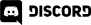# Home > Programming >

17. December 2012

This math recipe is a bit different.  It is in direct response to a question I received about how to give your sprite the ability to shoot.  That is exactly what we will be doing in this recipe.  There is no actual new math, it actually is just applying our prior recipes on Velocity and our recipe on Rotating to face a point.  As those prior recipes both explained how they work, this particular entry is going to be light on description.

## Just the Math

// calculate the speed relative to the mouse click distance

bullet.speed *= (bullet.y - bullet.targetY)/100;

// now calculate the angle between the bullet and mouse click

bullet.angle = Math.atan2(bullet.targetY - bullet.y, bullet.targetX - bullet.x );

bullet.angle = bullet.angle * (180/Math.PI);

bullet.onTick = function(delta){

var velocityX = Math.cos((bullet.angle) * Math.PI / 180* (bullet.speed * delta);

var velocityY = Math.sin((bullet.angle) * Math.PI / 180* (bullet.speed * delta);

bullet.x += velocityX;

bullet.y += velocityY;

}

### Controls:

Click anywhere on screen to shoot. The location determines the angle of the shot, while distance from the jet determines the speed

## Description

The actual description behind the mathematics are available at the two linked tutorials at the beginning and end of this recipe.  This is just a quick explanation of exactly what this application does.  We are creating an Array of bullet objects, a class we define ourselves inline.  The bullet simply contains its location (x,y), the location it is targeting (the user click position, targetX and targetY), the angle, speed ( pixels per second ) and finally a graphic that we will create in a moment.  We set the users speed using a multiple relative to the click distance away from the jet sprite.  For example, if the user clicks at 40 on the Y axis, the bullet be moving at  50 * (340-40)/100 == 50 * 3 == 150 pixels / second.  For the record, don't click at 340 in the Y axis or your bullet won't go anywhere! :)

Now that we have the speed, we calculate the initial angle between the jet and the location the user clicked.  Next we register an on tick() method that will be called each frame to update the bullet.  We simply check to see if the bullet is completely off screen in any direction, and if it is, we remove it from our array.  Otherwise we apply our velocity along our given angle, and update the X and Y values accordingly.  The remains of the tick function simply draw the newly updated bullet.

## Complete Code

<!DOCTYPE html>

<html>

<script src="http://code.createjs.com/easeljs-0.5.0.min.js"></script>

<script>

var jetSprite;

var stage;

var bullets;

function demo(){

bullets = new Array();

stage = new createjs.Stage("theCanvas");

stage.canvas.onmouseover = function(e){

document.body.style.cursor = "crosshair";

}

stage.canvas.onclick = function(e){

userClicked = true;

var bullet = {

x: 200,

y:340,

targetX:e.x,

targetY:e.y,

angle:0,

speed:50,

bulletGraphic:null

};

// calculate the speed relative to the mouse click distance

bullet.speed *= (bullet.y - bullet.targetY)/100;

// now calculate the angle between the bullet and mouse click

bullet.angle = Math.atan2(bullet.targetY - bullet.y, bullet.targetX - bullet.x );

bullet.angle = bullet.angle * (180/Math.PI);

bullet.onTick = function(delta){

if(this.x < -10 || this.x > stage.canvas.width+10 || this.y < -10 || this.y > stage.canvas.height+10){

stage.removeChild(this.bulletGraphic);

// Remove this bullet from the bullet list

var idx = bullets.indexOf(this);

bullets.splice(idx,1);

console.log(idx + " " + bullets.length);

//console.log("Bullet destroyed");

}

var velocityX = Math.cos((bullet.angle) * Math.PI / 180* (bullet.speed * delta);

var velocityY = Math.sin((bullet.angle) * Math.PI / 180* (bullet.speed * delta);

bullet.x += velocityX;

bullet.y += velocityY;

if(this.bulletGraphic !== null)

stage.removeChild(this.bulletGraphic);

var g = new createjs.Graphics();

g.setStrokeStyle(5);

g.beginStroke(createjs.Graphics.getRGB(255,0,0));

g.drawCircle(this.x,this.y,10);

this.bulletGraphic = new createjs.Shape(g);

}

bullets.push(bullet);

}

jetSprite = new createjs.Bitmap("jetsprite.small.png");

jetSprite.regX = 30// Half image width

jetSprite.regY = 40// Half image height

jetSprite.y = 360;

jetSprite.x = 200;

}

//And go...

stage.update();

// onFrame will be called each "tick". Default is 50ms, or 20FPS

}

function onFrame(elapsedTime) {

// Convert from milliseconds to fraction of a second

var delta = elapsedTime /1000;

for(var i = 0; i < bullets.length; i++){

bullets[i].onTick(delta);

}

stage.update();

}

</script>

<body>

<canvas width=400 height=400 id="theCanvas" style="background-color:black" />

</body>

</html>